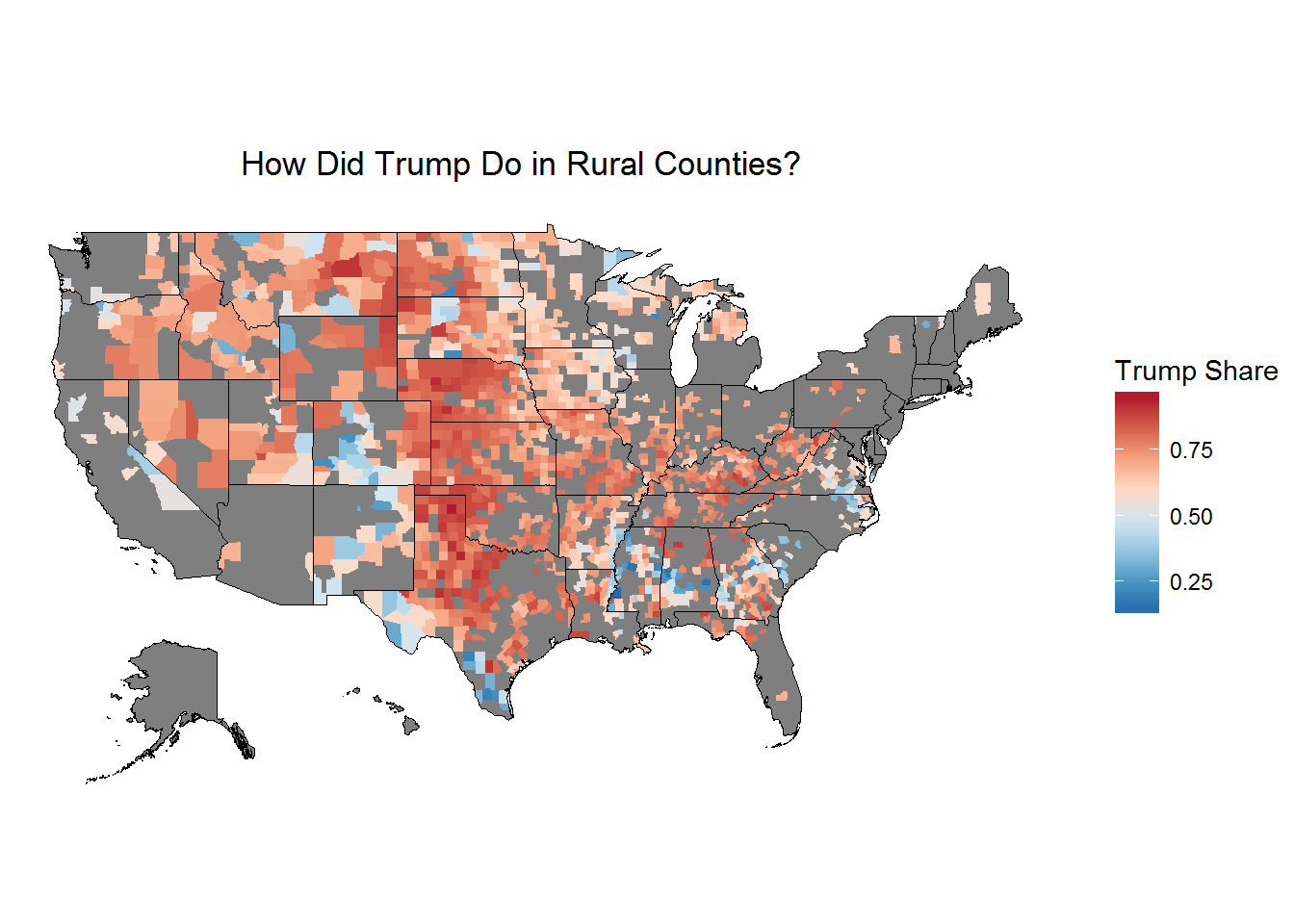``````library(ggplot2)
library(foreign)
library(gridExtra)
library(RColorBrewer)
library(choroplethr)
library(choroplethrMaps)
library(viridis)
library(dplyr)
library(DT)
library(knitr)``````

I got the county level voting datafile from here

I got the 2010 religious census data from here

``````vote <- read.csv("D:/2016_election/pres16results.csv", stringsAsFactors = FALSE)
vote\$fips <- gsub("(?<![0-9])0+", "", vote\$fips, perl = TRUE)
census <- read.dta("D:/2016_election/relcensus.dta", convert.factors = FALSE)
merge <- merge(census, vote, by=c("fips"))
merge <- merge(merge, suburbs, by=c("fips"))
pres <- filter(merge, cand_name == "Donald Trump" | cand_name == "Hillary Clinton")
trump <- filter(merge, cand_name == "Donald Trump")
clinton <- filter(merge, cand_name == "Hillary Clinton")
trump\$diff <- trump\$pct - clinton\$pct``````

## Rural Counties

There is no set definition of what rural means, so here’s what I did:

``````df <- select(trump, cntyname, stabbr, fips, votes, total, pct, POP2010, evanrate, code)
summary(df\$POP2010)``````
``````##    Min. 1st Qu.  Median    Mean 3rd Qu.    Max.
##      82   11310   26080   99010   67080 9819000``````

I am going to use the counties whose 2010 total population was below the mean of 26,080. Let’s see how Trump did in those counties.

``````rural <- filter(df, POP2010 <=26080)
rural\$region <- rural\$fips
rural\$value <- rural\$pct
palette_rev <- rev(brewer.pal(8, "RdBu"))
choro = CountyChoropleth\$new(rural)
choro\$title = "                         How Did Trump Do in Rural Counties?                                "
choro\$set_num_colors(1)
choro\$ggplot_polygon = geom_polygon(aes(fill = value), color = NA)
choro\$ggplot_scale = scale_fill_gradientn(name = "Trump Share", colours = palette_rev)
choro\$render()``````## Counties with Above Average Evangelicals

Let’s do the same with evangelicals.

``summary(df\$evanrate)``
``````##    Min. 1st Qu.  Median    Mean 3rd Qu.    Max.    NA's
##     0.0   107.7   190.5   233.8   334.1  1309.0      18``````

The median here is 190.5 evangelicals per 1000. So any county that’s 19.1% evangelical is in this subset.

``````high_evan <- filter(df, evanrate >= 190.5)
high_evan\$region <- high_evan\$fips
high_evan\$value <- high_evan\$pct
palette_rev <- rev(brewer.pal(8, "RdBu"))
choro = CountyChoropleth\$new(high_evan)
choro\$title = "                                   Counties with Above Average Evangelicals"
choro\$set_num_colors(1)
choro\$ggplot_polygon = geom_polygon(aes(fill = value), color = NA)
choro\$ggplot_scale = scale_fill_gradientn(name = "Trump Share", colours = palette_rev)
choro\$render()``````